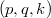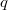﻿

### On Defining the$(p,q,k)$-Generalized Gamma Function

#### Abstract

In this paper, we introduce a$(p,q,k)$-generalization of the gamma function and investigate some basic identities and properties. Further, we define a$q$-integral representation for this function. As an application, we give some double inequalities concerning the$(p,q,k)$-generalized gamma function by using of its$q$-integral representation.

DOI Code: 10.1285/i15900932v39n1p107

Keywords: Gamma function; \$q\$-integral; \$(p,q,k)\$-generalized gamma function; \$(p,q,k)\$-generalized Pocchammer symbol; inequality

Full Text: PDF